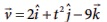# UNIT II: Kinematics - Online Test

Q1. Which one of the following Cartesian coordinate systems is not followed in physics?
Explaination / Solution:
No Explaination.

Q2. Identify the unit vector in the following.
Explaination / Solution:
No Explaination.

Q3. Which one of the following physical quantities cannot be represented by a scalar?
Explaination / Solution:
No Explaination.

Q4. Two objects of masses m1 and m2 fall from the heights h1 and h2 respectively. The ratio of the magnitude of their momenta when they hit the ground is
Explaination / Solution:
No Explaination.

Q5. If a particle has negative velocity and negative acceleration, its speed
Explaination / Solution:
No Explaination.

Q6. If the velocity is, then the magnitude of acceleration at t = 0.5 s is
Explaination / Solution:
No Explaination.

Q7. If an object is dropped from the top of a building and it reaches the ground at t = 4 s , then the height of the building is (ignoring air resistance) (g = 9.8 ms–2)
Explaination / Solution:
No Explaination.

Q8. A ball is projected vertically upwards with a velocity v. It comes back to ground in time t. Which v-t graph shows the motion correctly?
Explaination / Solution:
No Explaination.

Q9. If one object is dropped vertically downward and another object is thrown horizontally from the same height, then the ratio of vertical distance covered by both objects at any instant t is
Explaination / Solution:
No Explaination.

Q10. A ball is dropped from some height towards the ground. Which one of the following represents the correct motion of the ball?
Explaination / Solution:
No Explaination.